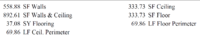# Calculate Height of Room

#### kenshepco

##### New member
can you determine the height of a room if you know the square footage of the walls and SF of the floor? Assume the ceiling height is the same in all of the room.

#### Subhotosh Khan

##### Super Moderator
Staff member
can you determine the height of a room if you know the square footage of the walls and SF of the floor? Assume the ceiling height is the same in all of the room.
Is the room made of rectangular floor and rectangular walls?

With or without doors/windows/skylights?

Please show us what you have tried and exactly where you are stuck.

Please follow the rules of posting in this forum, as enunciated at:

#### Dr.Peterson

##### Elite Member
can you determine the height of a room if you know the square footage of the walls and SF of the floor? Assume the ceiling height is the same in all of the room.
Do you know each wall's area, or only the total of all four walls? Five numbers (actually three) should be sufficient to determine the three dimensions of the room, but two would not be.

#### kenshepco

##### New member
Do you know each wall's area, or only the total of all four walls? Five numbers (actually three) should be sufficient to determine the three dimensions of the room, but two would not be.
Thank you very much for the reply. All I have is the SF of the Floor (Ceiling, which is the same as the floor) and the total SF of the Walls. I am really trying to determine the cubic feet of the room, which is the SF of the Floors X the height, however, I don't know the height. Therefore, I was trying to determine the height, so that I could multiply it times the SF of the Floors. So, I assume if I only have the two numbers (SF of Walls and SF of Floor), that I cannot determine the height?

#### kenshepco

##### New member
Thank you very much for the reply. All I have is the SF of the Floor (Ceiling, which is the same as the floor) and the total SF of the Walls. I am really trying to determine the cubic feet of the room, which is the SF of the Floors X the height, however, I don't know the height. Therefore, I was trying to determine the height, so that I could multiply it times the SF of the Floors. So, I assume if I only have the two numbers (SF of Walls and SF of Floor), that I cannot determine the height?

Just to add another note, here is exactly what I have. I am trying to determine cubic feet of the room.#### Subhotosh Khan

##### Super Moderator
Staff member
Just to add another note, here is exactly what I have. I am trying to determine cubic feet of the room.
View attachment 23257
What about Windows and doors ? How are those accounTed for in the sq.footage?

#### Dr.Peterson

##### Elite Member
Thank you very much for the reply. All I have is the SF of the Floor (Ceiling, which is the same as the floor) and the total SF of the Walls. I am really trying to determine the cubic feet of the room, which is the SF of the Floors X the height, however, I don't know the height. Therefore, I was trying to determine the height, so that I could multiply it times the SF of the Floors. So, I assume if I only have the two numbers (SF of Walls and SF of Floor), that I cannot determine the height?
If you only had the two areas, that would not be enough. For example, if you only had floor = 333.73 and walls = 558.88 (and assuming those are not reduced by doors and windows, and everything is rectangular), then you can say only that LW=333.73 and 2(L+W)H = 558.88, which couldn't be solved for each variable. (Note that wall area equals floor perimeter times height, because it's the sum of each side times the height.)

But ...
Just to add another note, here is exactly what I have. I am trying to determine cubic feet of the room.
View attachment 23257
It appears that you also have the perimeter of the floor, so you can say 2L+2W = 69.86. This is a third equation, and gives you the missing information. We can conclude that H = 558.88/69.86 = 8 feet exactly. Therefore, the volume of the room is area times height = 333.73*16 = 5339.68 cubic feet.

#### kenshepco

##### New member
Thank you very much. It appears that you solved it. However, wouldn't the cubic feet be the SF of the Floor (L * W) * H, or 333.73 * 8 = 2,669.84, not 333.73 * 16

#### Dr.Peterson

##### Elite Member
Thank you very much. It appears that you solved it. However, wouldn't the cubic feet be the SF of the Floor (L * W) * H, or 333.73 * 8 = 2,669.84, not 333.73 * 16
Yes, I think the 16 was left over from an earlier error and I forgot to correct it.